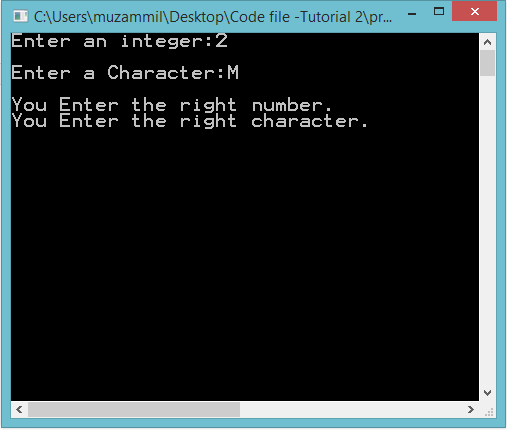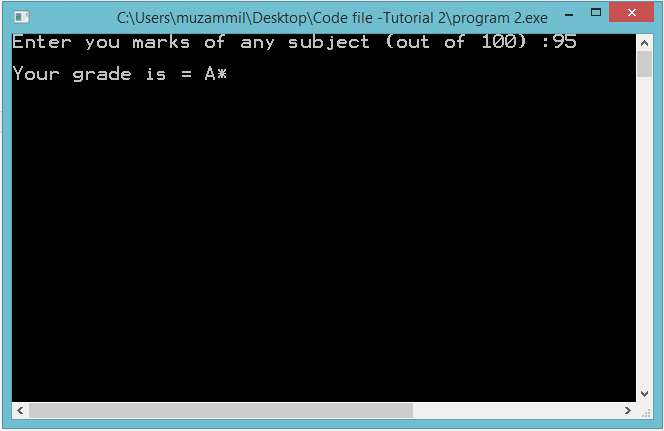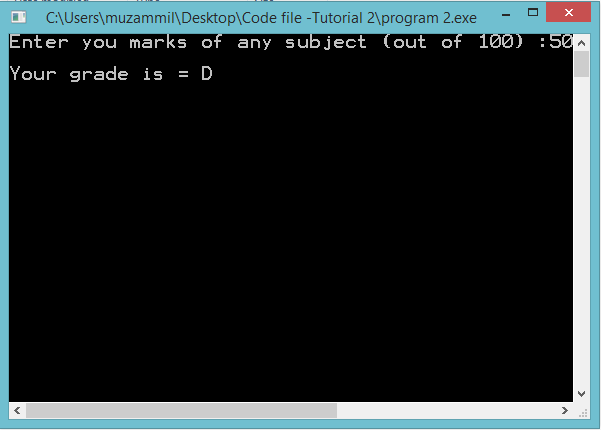# Conditional Statements

### Conditional Statements

In this part you will learn: 1. C syntax 2. Conditional Statements 3. Operators In this tutorial I will teach you about conditional statements in C. The first program will be a basic one with a most basic conditional statement. The second one is a grade calculator. First Program. Basic Conditional Statements Basic Step: Open Dev C++ then File > new > source file and start writing the code below.
`#include<stdio.h>#include<conio.h>`
These two are the most common and basic header files. The first one stdio.h is used to grant us most of the basic functions of C like Input and Output functions. The second one conio.h provides us functions like getch() which is used to pause our screen until we press a button.
`int main(){	int a;	char c;	printf("Enter an integer:");	scanf("%d",&a); 	printf("\nEnter a Character:");	scanf(" %c",&c);`
Now at first we declared two variables. A integer variable and a character variable. We took the input from the user in these variables using scanf() function.
`	if(a==2)	{		printf("\nYou Enter the right number.");	}        else	{		printf("\nYou Enter the wrong number.");	} 	if(c=='M')	{		printf("\nYou Enter the right character.");	}	else	{		printf("\nYou Enter the wrong character.");	}  	getch();	} `
This is the conditional statement i.e ‘if()’ and ‘else’ . We write our condition in brackets and in square brackets we write the code that we want to execute when our condition in if() is met. Like in this program I have placed a condition that if a is equal to 2 then the program should output a statement “ you entered the right number.” And if a is not equal to 2 then the ‘else’ part will execute and the program will output a statement “ you entered the wrong number.”. only one condition executes at a time either ‘if’ or else part. In the condition we enter ‘==’ this is the operator in C which means ‘equal to’ similar to ‘=’ in mathematics. Same is the condition with the character variable too. Execute > compile then Execute > run OutputGrade Calculator Basic Step: Open Dev C++ then File > new > source file and start writing the code below.
`#include<stdio.h>#include<conio.h>`
These two are the most common and basic header files. The first one stdio.h is used to grant us most of the basic functions of C like Input and Output functions. The second one conio.h provides us functions like getch() which is used to pause our screen until we press a button.
`int main(){	int m; 	printf("Enter you marks of any subject (out of 100) :");	scanf("%d",&m);`
At first we just declared an integer variable. Then afterwards we took input from the user in our integer variable. The user should enter his marks of any subject out of 100.
`if(m>=90 && m<=100)	{		printf("\nYour grade is = A*");	}	else if(m>=80 && m<=90)	{		printf("\nYour grade is = A");	} 	else if(m>=70 && m<=80)	{		printf("\nYour grade is = B");	}	else if(m>=60 && m<=70)	{		printf("\nYour grade is = C");	}	else if(m>=50 && m<=60)	{		printf("\nYour grade is = D");	}	else if(m>=45 && m<=50)	{		printf("\nYour grade is = E");	}	else if(m<45 && m>=0)	{		printf("\nYou are Fail");	}	else	{		printf("\nInvalid number");	} 	getch();}`
In this part of code me place many statements. These statement compare the marks of the student with the grading criteria that we have set. You can set any condition you like. The program first checks the marks of the students with first condition. If it is a match with condition the program executes the code in the brackets and ends the program. Otherwise it checks the ‘else if() ‘ conditions. Note that only one statement executes in the whole program. It can be either if() or else if or else. In the conditions we have used few operators that might be new to you. The first is ‘>=’ operator this means “greater or equal to”. The second is ‘ compile then Execute > run Output